# Regular Polygons

Polygons are plane (flat or 2-Dimensional) shapes that have straight sides and pointy angles.

A polygon is regular if all of its sides have the same length, and all of its angles have the same size.

Let's have a look at some of the common regular polygons;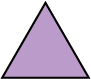The triangle in the picture is a regular polygon.

It has three sides and three angles.

All of its sides have the same length, and all of its angles are the same size.

Not all triangles are regular. Another name for a regular triangle is an equilateral triangle.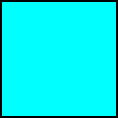A square is a regular polygon.

It has four sides and four angles.

Another name for a polygon with four sides is a quadrilateral. So, a square is a regular quadrilateral.

All of its sides have the same length, and all of its angles are right angles.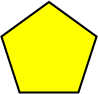The polygon in the picture is a regular pentagon.

It has five sides and five angles.

All of its sides have the same length, and all of its angles are the same size.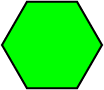The polygon in the picture is a regular hexagon.

It has six sides and six angles.

All of its sides have the same length, and all of its angles are the same size.

Bees build their honeycomb out of regular hexagons.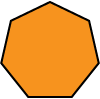The polygon in the picture is a regular heptagon.

It has seven sides and seven angles.

All of its sides have the same length, and all of its angles are the same size.The polygon in the picture is a regular octagon.

It has eight sides and eight angles.

All of its sides have the same length, and all of its angles are the same size.The polygon in the picture is a regular nonagon.

It has nine sides and nine angles.

All of its sides have the same length, and all of its angles are the same size.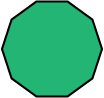The polygon in the picture is a regular decagon.

It has ten sides and ten angles.

All of its sides have the same length, and all of its angles are the same size.

You can build regular polygons with as many sides as you like. All the sides must have the same length, and all the angles must be equal.

## Other Commonly Used Polygons that are Not Regular

We use lots of different polygons that aren't regular all the time. Let's have a look at some quadrilaterals (4-sided polygons) that aren't regular. Some of their sides might have different lengths and/or some of their angles might have different sizes.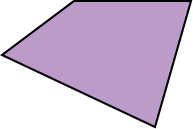The quadrilateral (4-sided shape) in the picture is as far away from regular as possible.

It has four sides and four angles.

None of its sides have the same length, and none of its angles are the same size.This rectangle is not a regular polygon.

It has four sides and four angles.

All of its angles are right angles, but it has sides of two different lengths.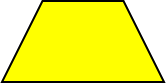This is a trapezium.

This particular trapezium is a special type with one pair of sides that have equal length. It also has two pairs of equal angles.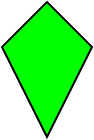A kite is also a quadrilateral.

It has two pairs of sides that are equal to each other, and one pair of angles that are equal to each other.

## Some Common 2-dimensional Shapes that are not Polygons

These shapes are not polygons because they have curves on their boundaries. Many shapes like this exist. These are two of the most common ones.This shape is a circle.

It is the shape you would trace out if you stood in one place and swung a tennis ball, wrapped in a stocking, around your head.

There are many ways to fold a circle in half.This shape is an oval.

This particular type of oval is called an ellipse, but there are others that aren't quite so symmetric.

It has two lines of symmetry: there are two different ways to fold it in half.

### Description

This mini book covers the core of Math for Foundation, Grade 1 and Grade 2 mathematics including

1. Numbers
3. Subtraction
4. Division
5. Algebra
6. Geometry
7. Data
8. Estimation
9. Probability/Chance
10. Measurement
11. Time
12. Money
13. and much more

This material is provided free of cost for Parent looking for some tricks for their Prekinder, Kinder, Prep, Year 1 and Year 2 children

### Learning Objectives

These lessons are for kids aged 4-8 with the core objective to expose their brains to concepts of addition, subtraction, division, algebra and much more.

Author: Subject Coach
You must be logged in as Student to ask a Question.